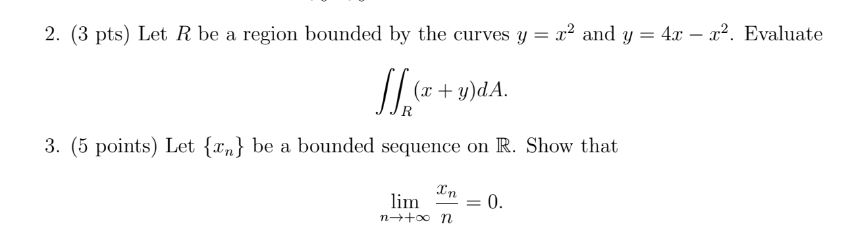asistioacer 2022-02-24 AnsweredPlease answer with solution.

You can still ask an expert for help

• Questions are typically answered in as fast as 30 minutes

Solve your problem for the price of one coffee

• Math expert for every subject
• Pay only if we can solve italenahelenash

2. Since $y={x}^{2}$ and $y=4x-{x}^{2}$

${x}^{2}=4x-{x}^{2}$

$2{x}^{2}-4x=0⇒2x\cdot \left(x-2\right)=0$

$2x=0⇒x=0$

since $x=0⇒y={x}^{2}⇒y=0$

$A=\left(0,0\right)$

$x-2=0⇒x=2$

since $x=2⇒y={x}^{2}⇒y=4$

$B=\left(2,4\right)$

if we take $dx$ slice (that mean the Area revolving about x-axis)

The interval of the integral become

$x\in \left[0,2\right]$

now let set up the integral of Area

$A={\int }_{a}^{b}{y}_{2}-{y}_{1}\ast dx$

$A={\int }_{0}^{2}\left(4x-{x}^{2}\right)-\left({x}^{2}\right)\cdot dx$

$A={\int }_{0}^{2}4x-2{x}^{2}\cdot dx={\left[2{x}^{2}-\frac{2}{3}{x}^{3}\right]}_{0}^{2}$

$\left[8-\frac{16}{3}\right]=\frac{24-16}{3}=\frac{8}{3}=2.667{\left(unite\right)}^{2}$